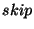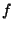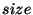Next: Using FFTEASY in Your Up: Using FFTEASY Previous: Using FFTEASY

## Overview of the Functions and Their Arguments

Here then are the four functions included in FFTEASY. I describe below what all the arguments are.

1. fftc1(float, int, int, int). FFT of a one-dimensional set of complex data.
2. fftcn(float, int, int, int). FFT of an ndims dimensional set of complex data.
3. fftr1(float, int, int). FFT of a one-dimensional set of real data.
4. fftrn(float, float, int, int, int). FFT of an ndims dimensional set of real data.

All of these functions take an arrayof the data to be transformed and an integerspecifying whether to take a forward transform () or an inverse transform (). The one-dimensional routines take an integergiving the number of data points in the array. For fftr1is the number of real data points while for fftc1is the number of complex data points, meaning that for fftc1should be an array of floats of size. The n dimensional routines take an integergiving the number of dimensions and an array of integersgiving the number of data points (real or complex) in each dimension. For the complex routines the data should alternate between real and imaginary parts, i.e.. Note that the last dimension of the arrayshould always be twice as large as the actual number of data points to account for the fact that it takes two floats to represent a complex number. (See the examples in section 4). Finally the function fftrn takes an additional arrayfor holding the Nyquist frequency components of the transform. I explain this below when I describe the storage scheme for the results of transforms of real data.

Note that even fftcn and fftrn take as input one dimensional arrays. Actually the functions assume that the data are arranged as in an n-dimensional array, so all you have to do is take your multidimensional array and cast it as a (float *) when you pass it to FFTEASY.Next: Using FFTEASY in Your Up: Using FFTEASY Previous: Using FFTEASY